# Intermediate maths solutions for Parabola

Intermediate Mathematics IIB solutions for Parabola textbook exercise 3(a) and 3(b) are given.

These solutions are very easy to understand. Study the textbook lesson very well. Then observe the example problems and solutions. Try them.

Observe the given Inter maths 2b Parabola solutions. Try them in your own method.

You can also see

SSC Maths text book Solutions class 10

Inter Maths 1A Solutions textbook

Inter Maths 1B textbook solutions

Inter Maths 1IA text book solutions

Inter Maths IIB text book solutions

M

# Inter maths solutions for ParabolaExercise 3(a)

Chapter 3 – Parabola

Exercise 3(a)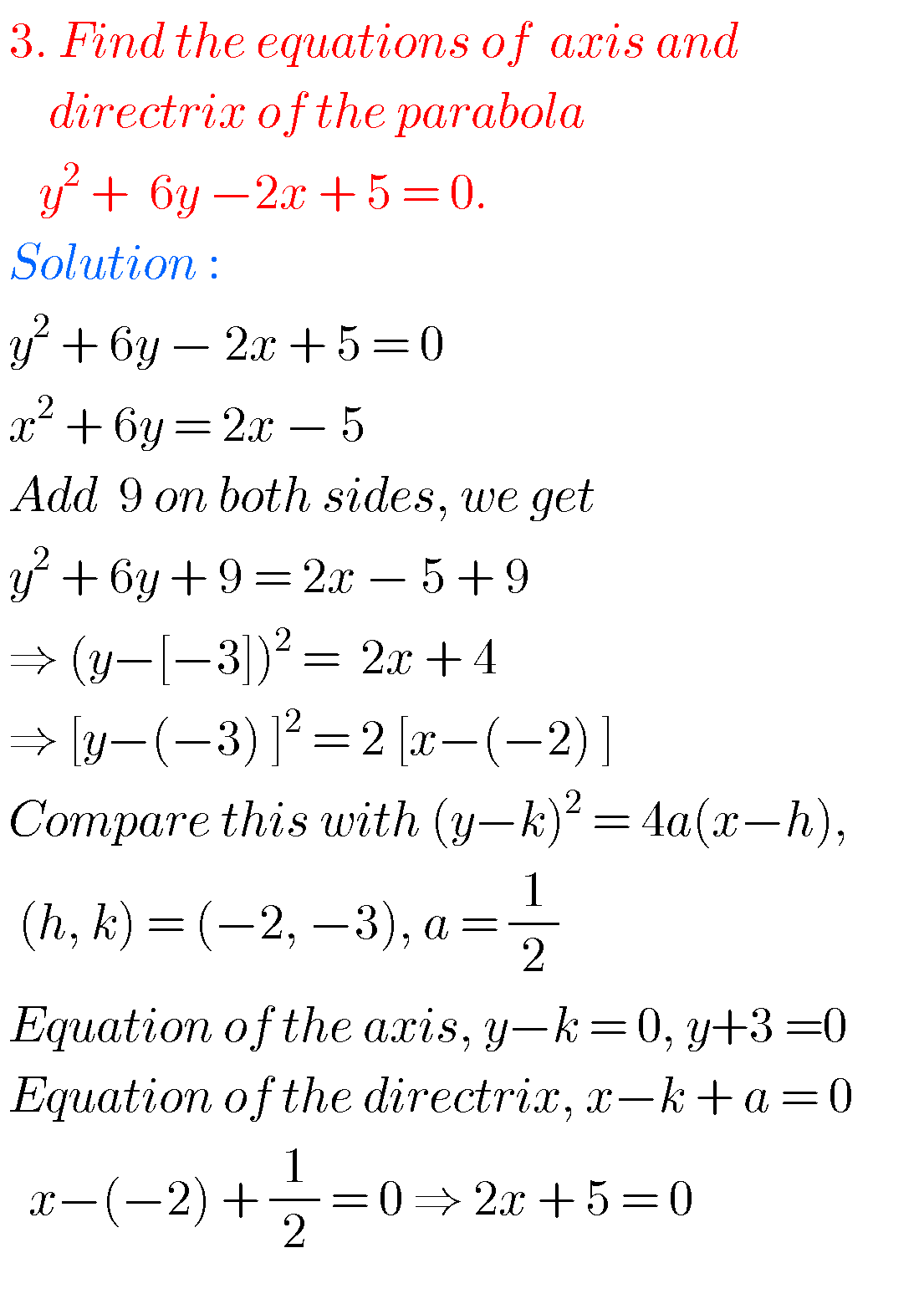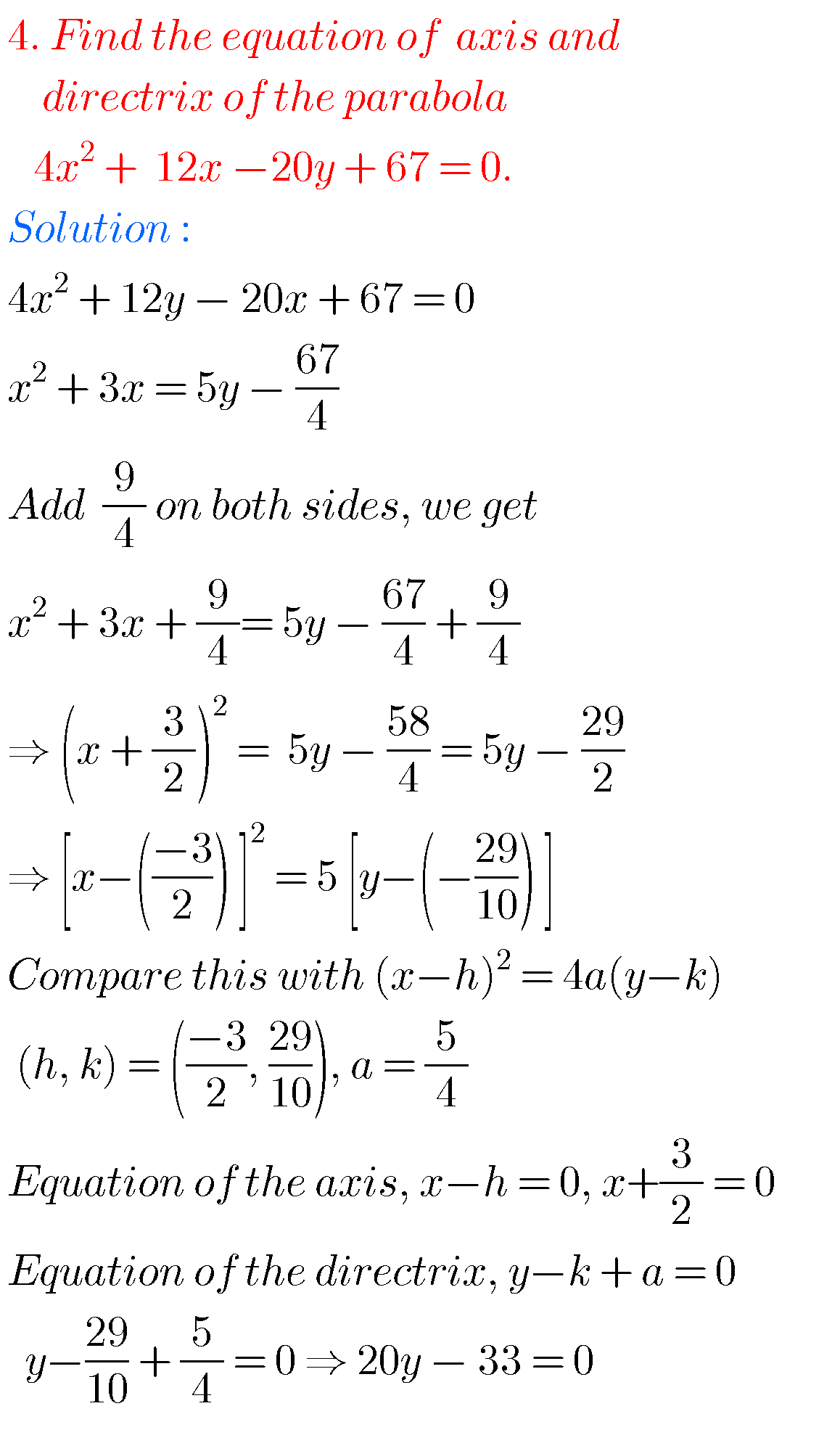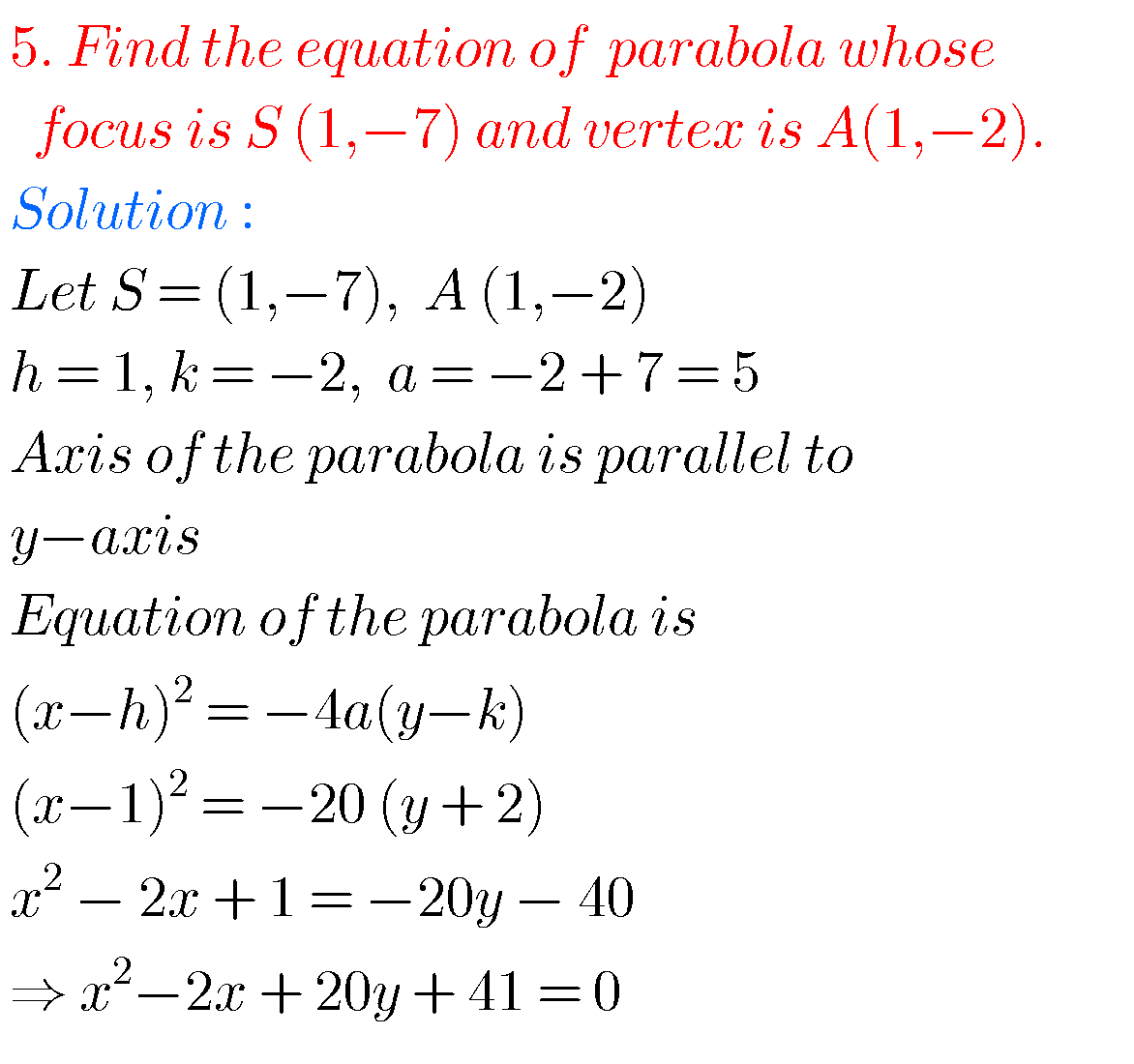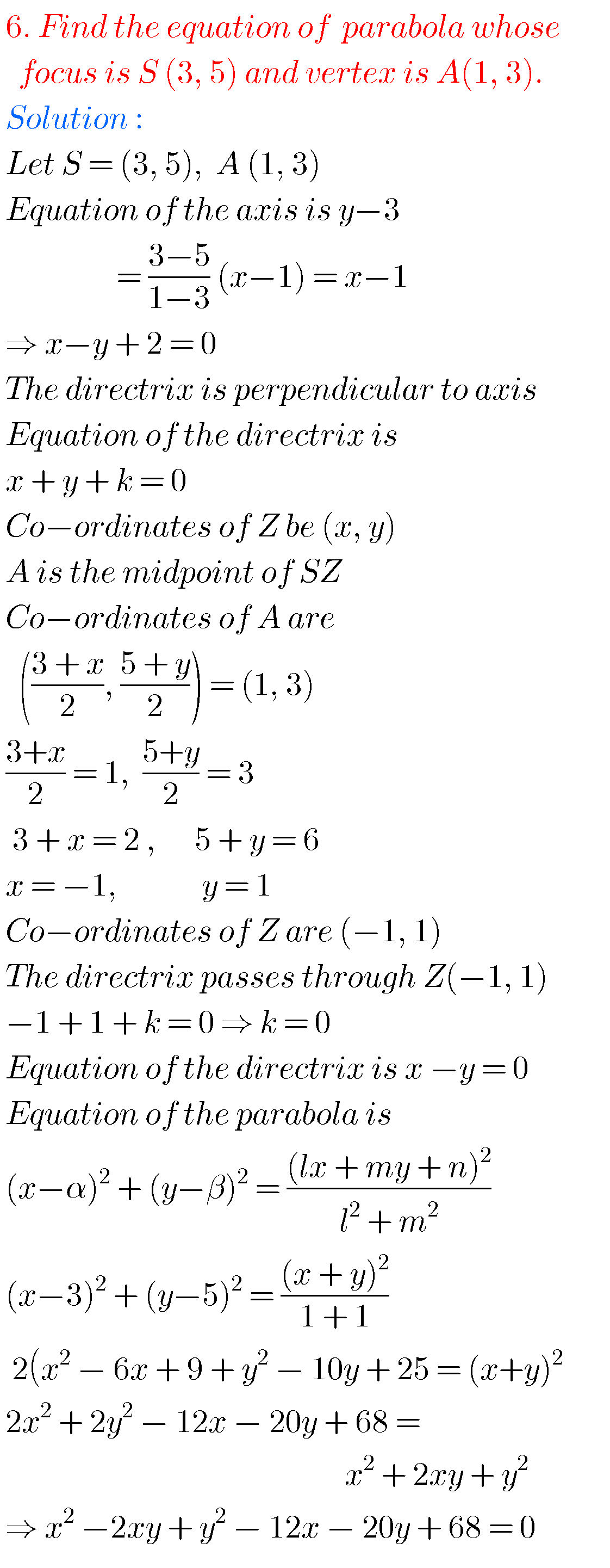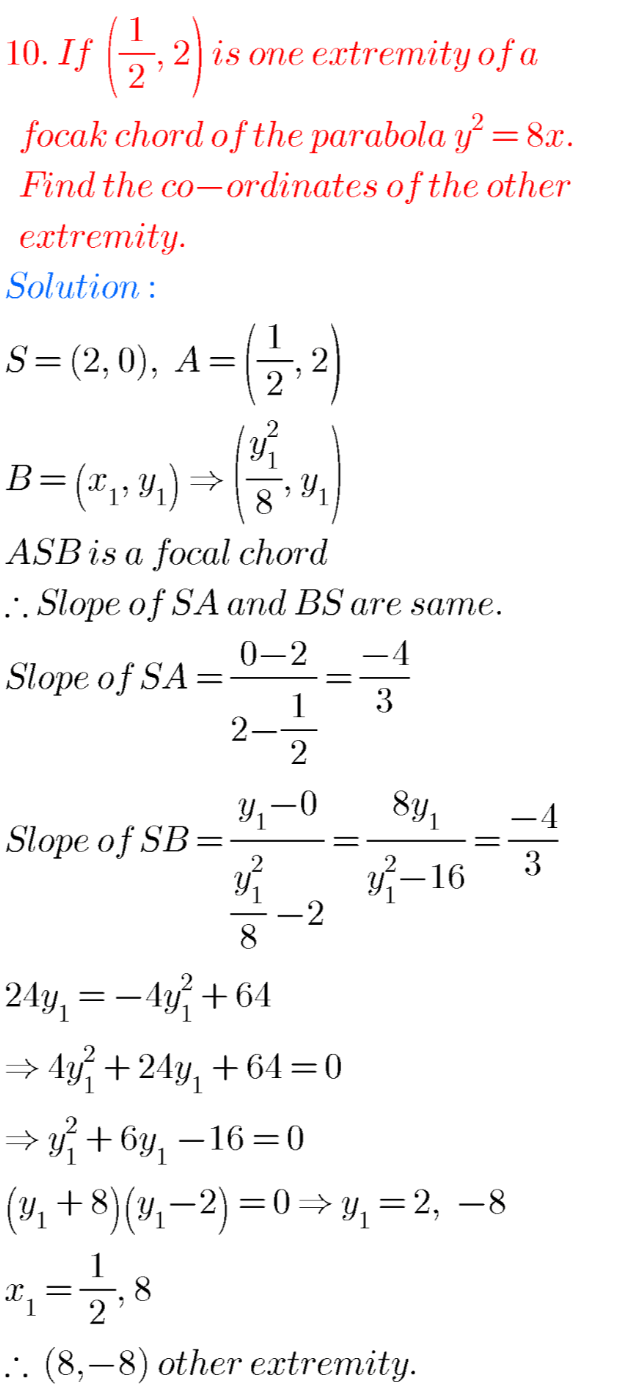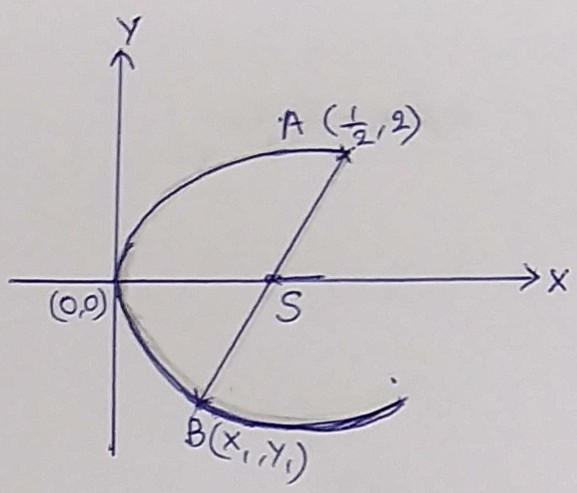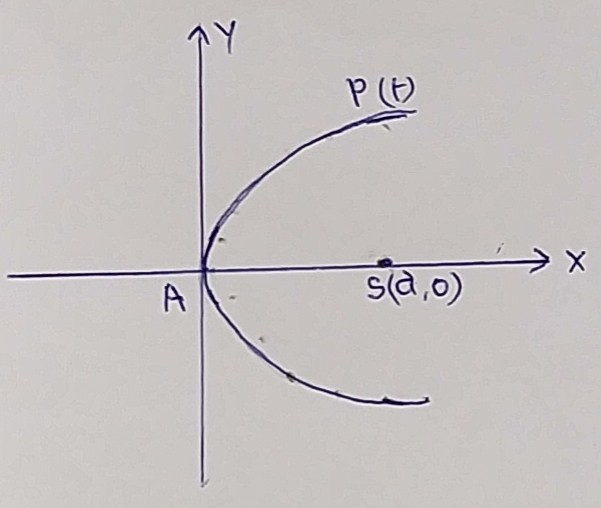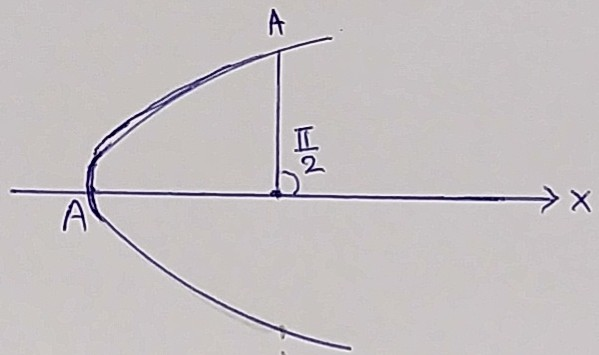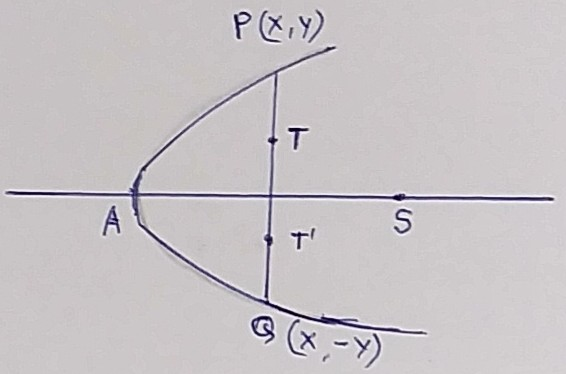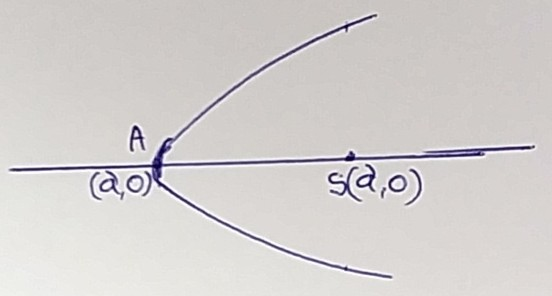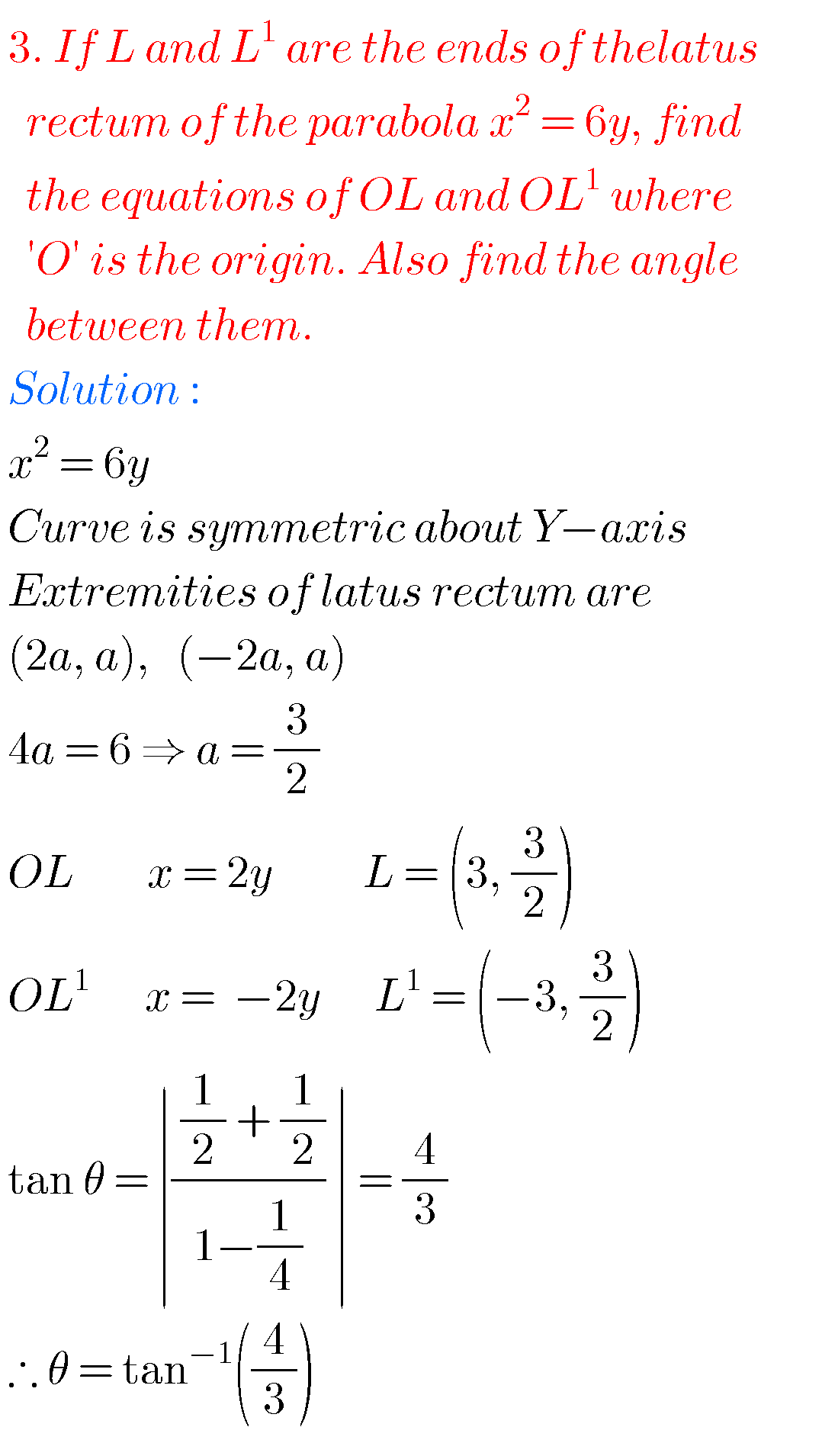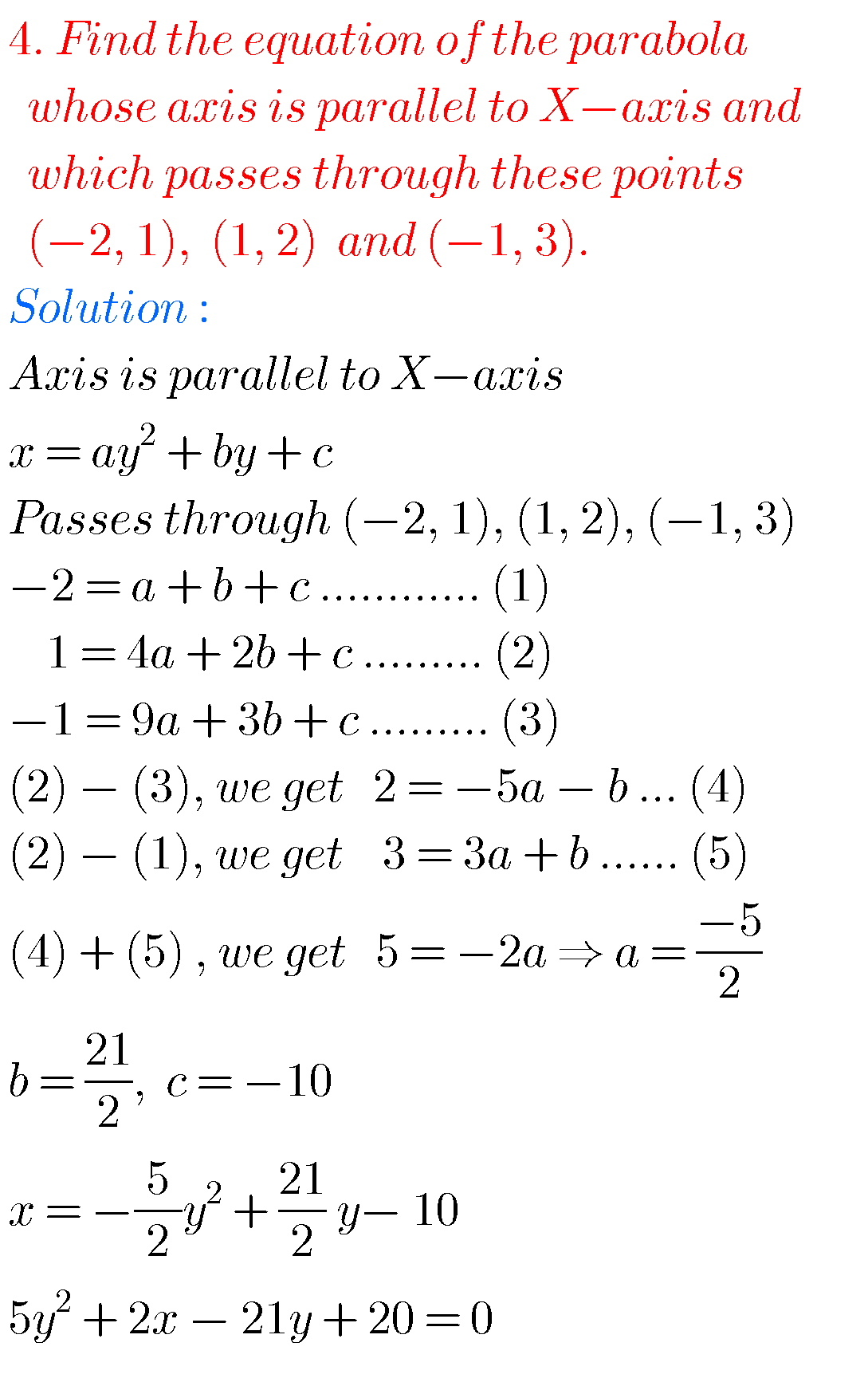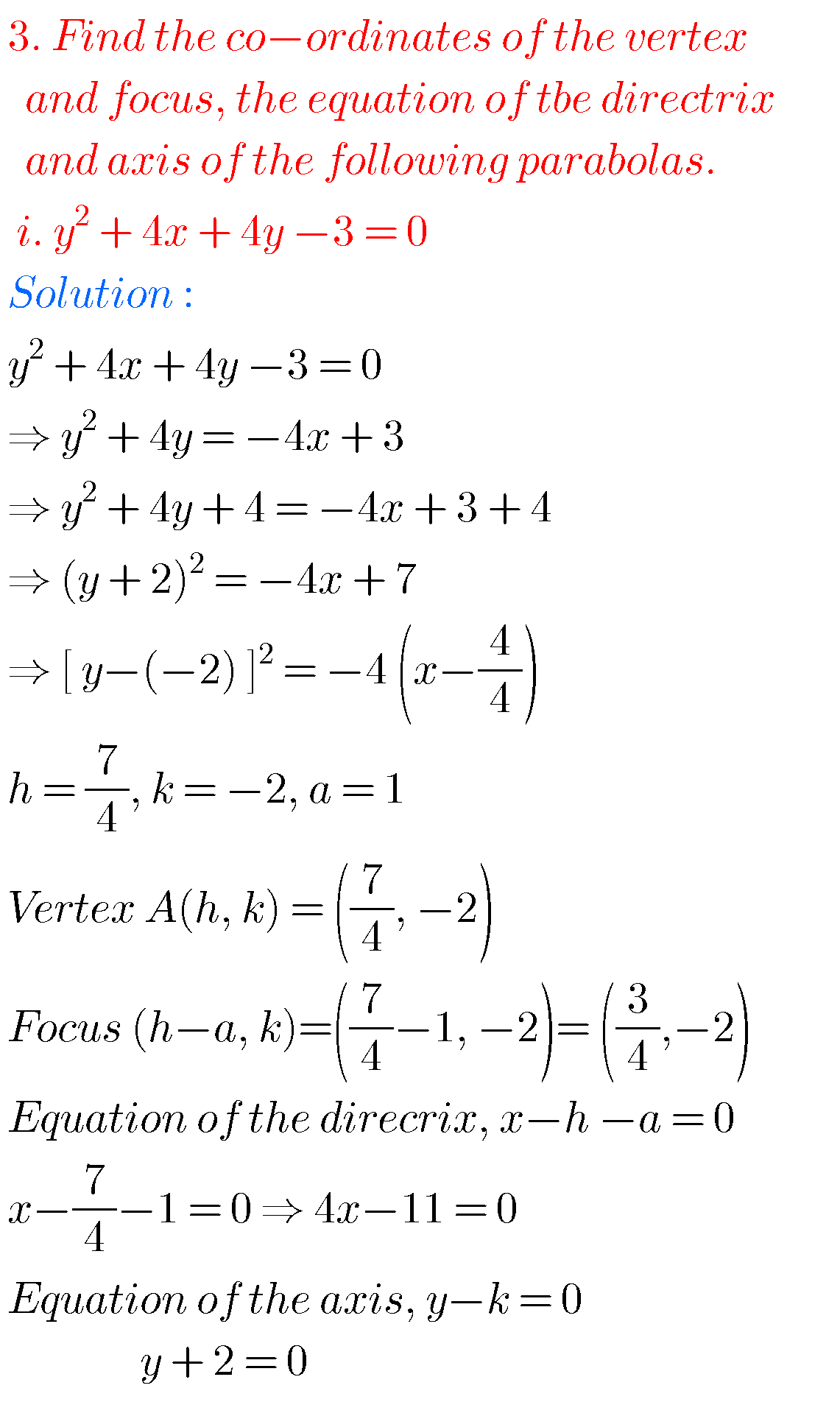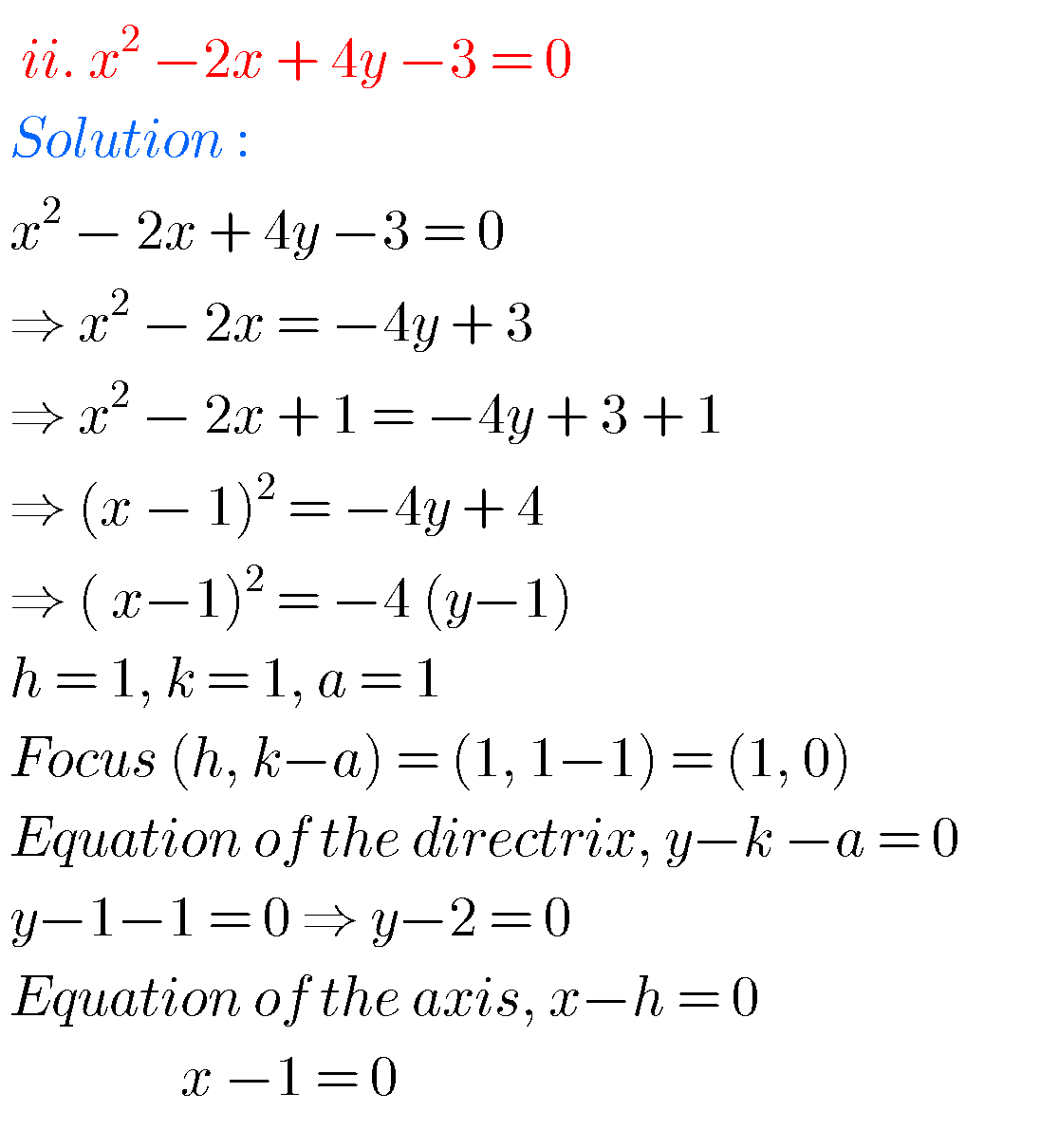## Maths solutions for Inter Parabola

Exercise 3(b)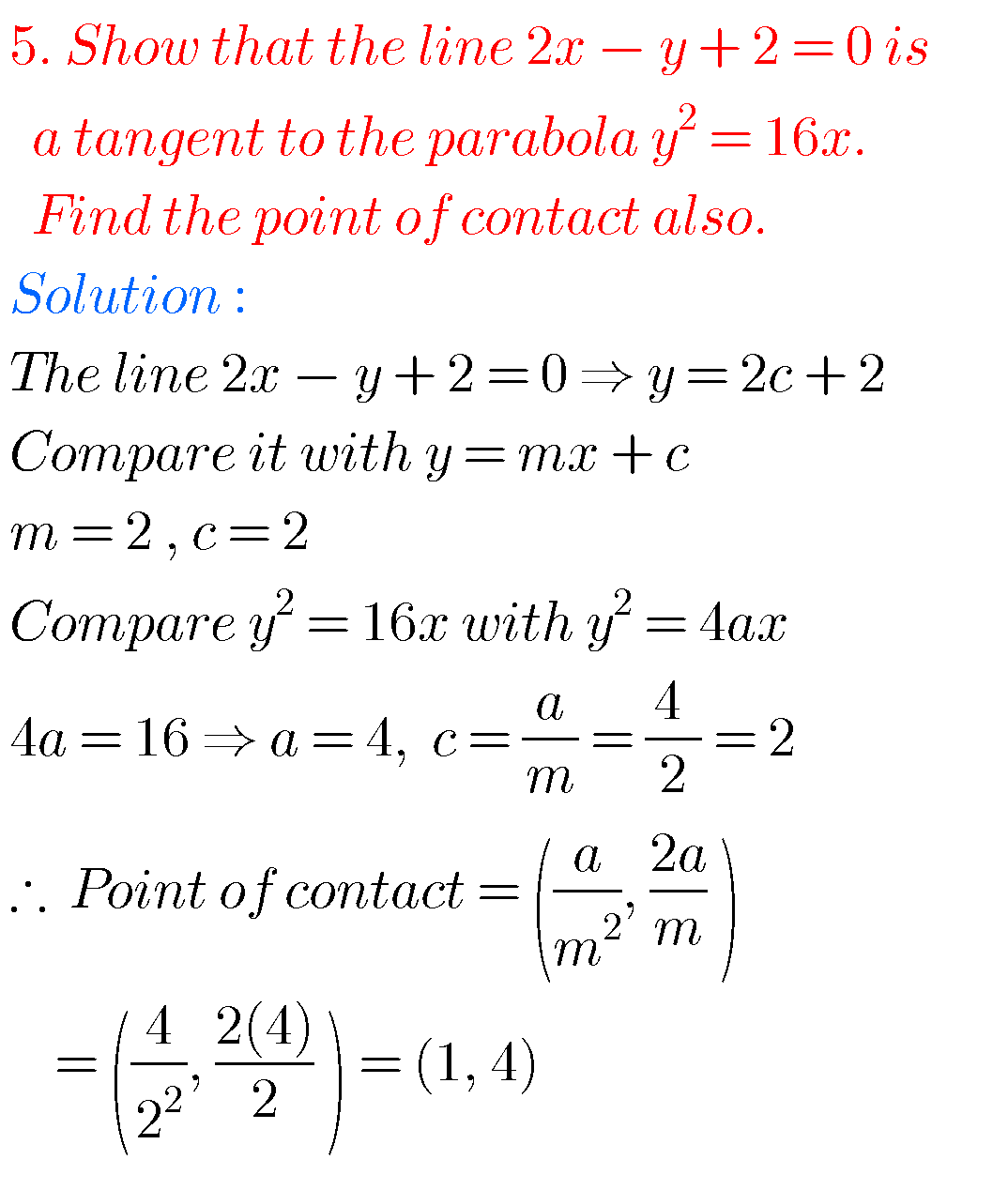### Parabola solutions Inter maths 2b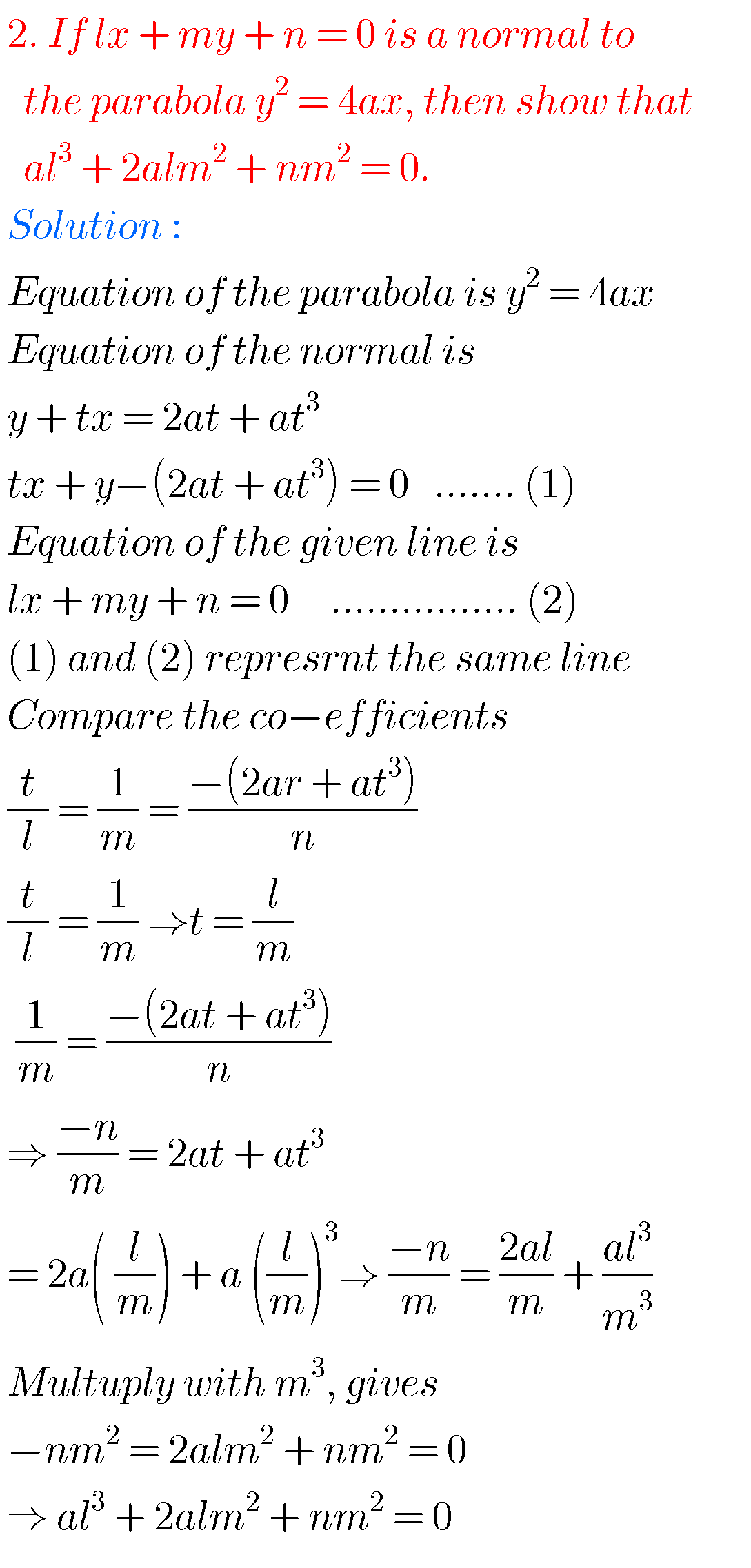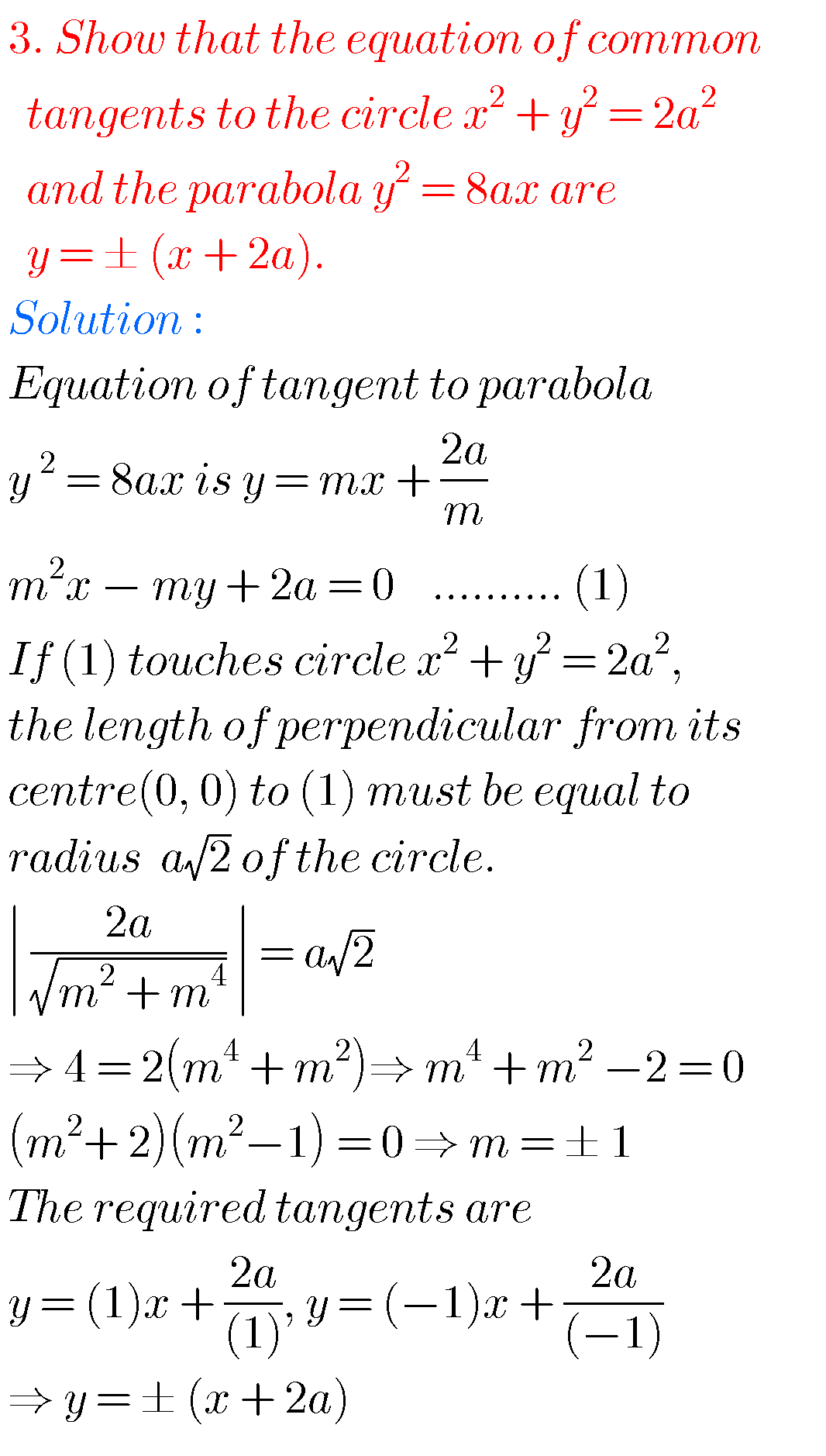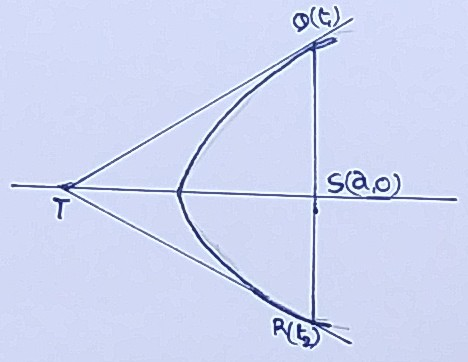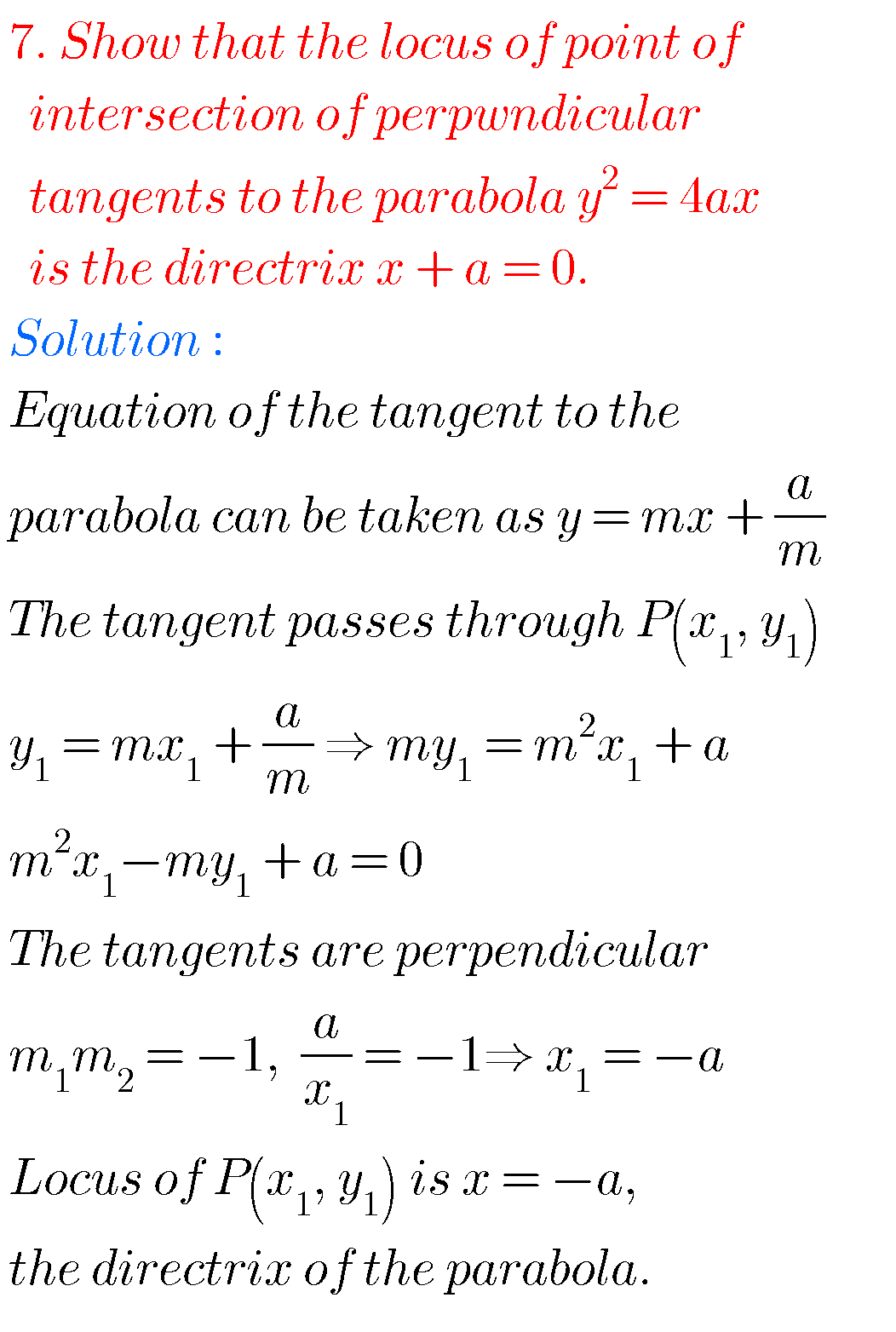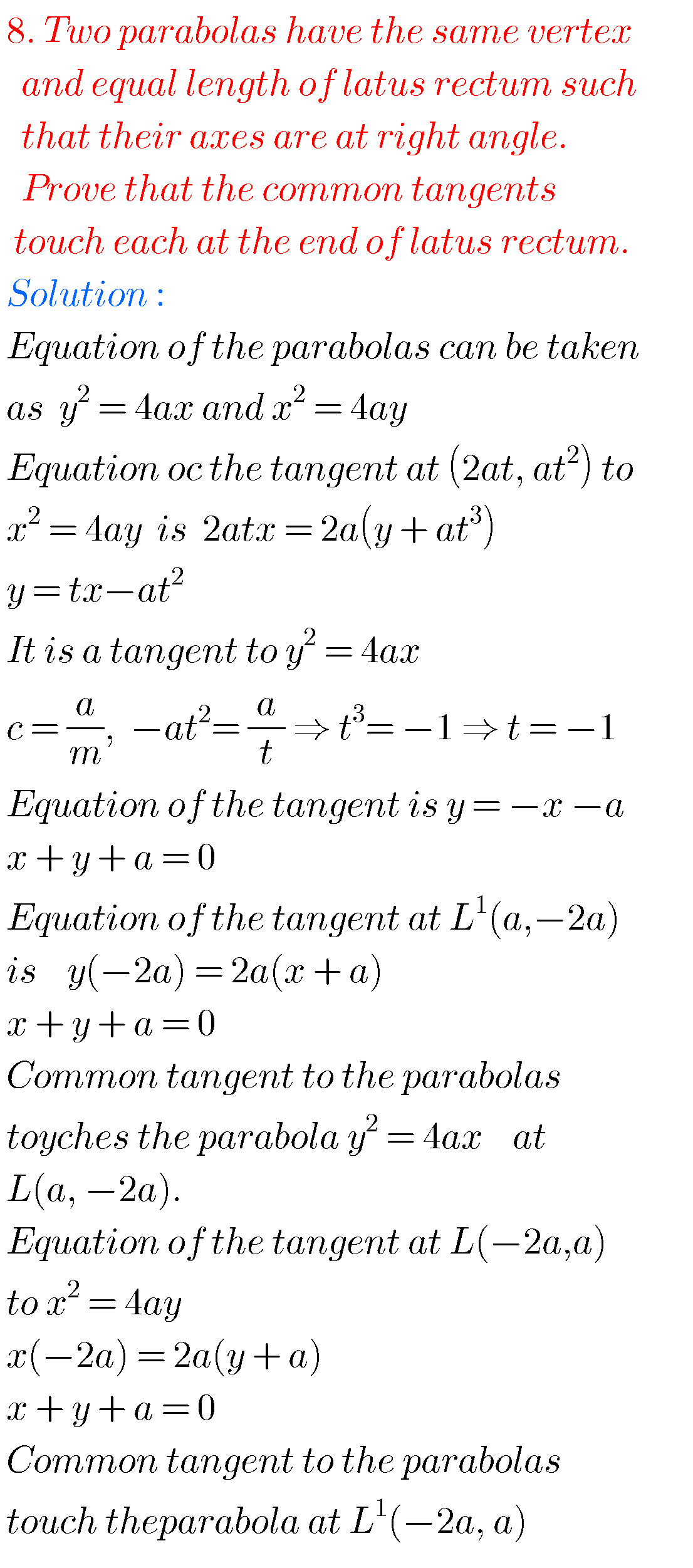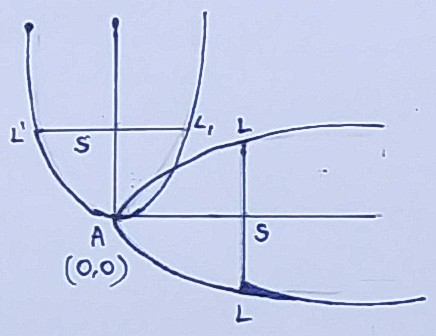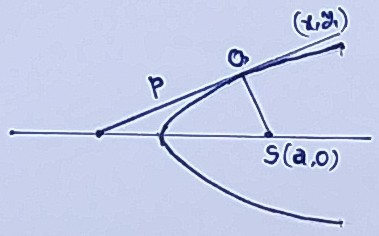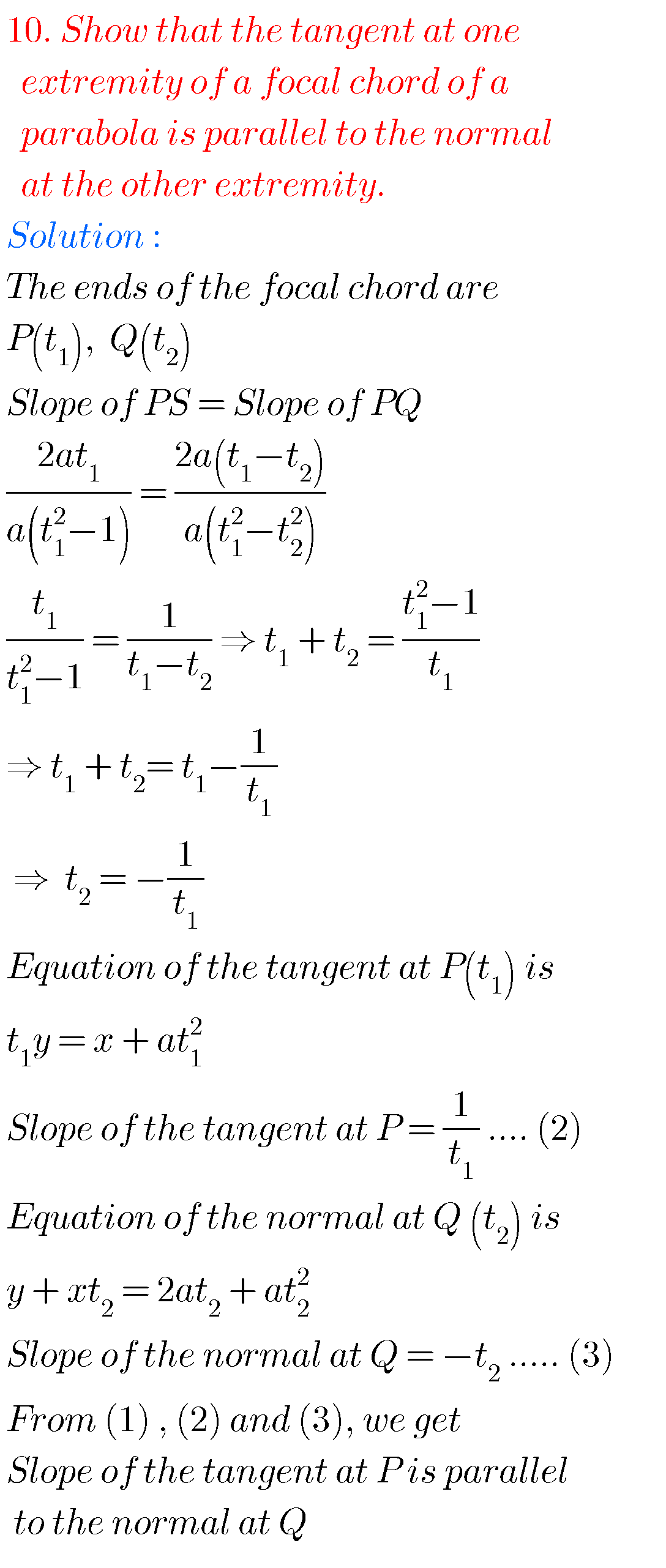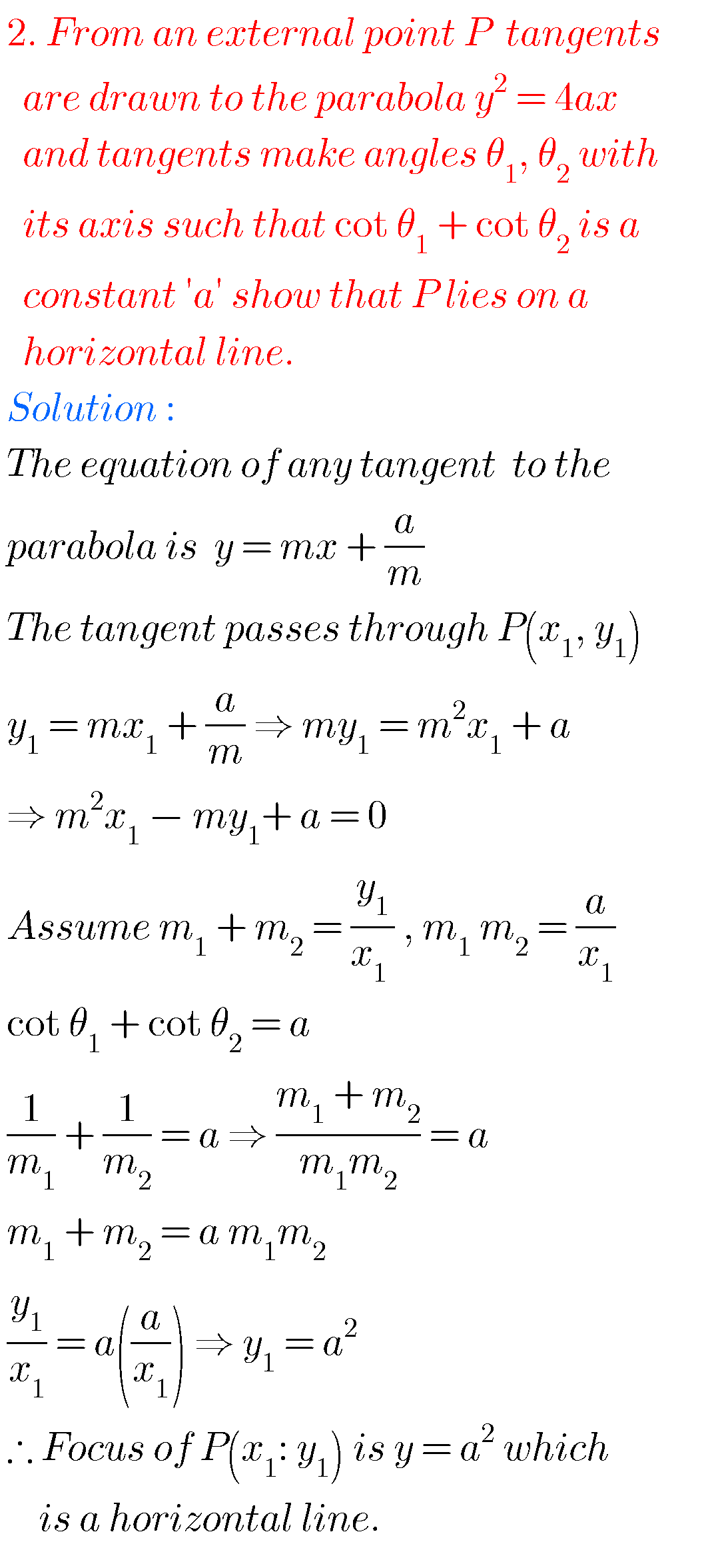Note : Observe the solutions and try them in your own method.